您可以捐助，支持我们的公益事业。 1元 10元 50元 认证码：必填求知 文章 文库 Lib 视频 iProcess 课程 认证 咨询 工具 讲座 Modeler Code要资料订阅捐助
Python 机器学习实战教程：回归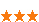2728 次浏览     评价： 好 中 差

 pip install numpy pip install scipy pip install scikit-learn pip install matplotlib pip install pandas

 pip install quandl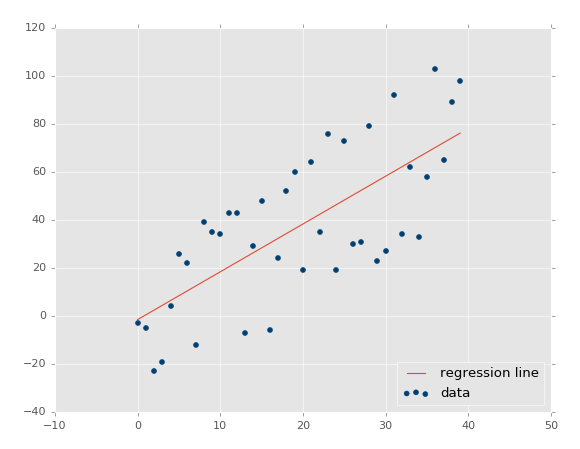import pandas as pd import quandl df = quandl.get("WIKI/GOOGL") print(df.head())

 Open High Low Close Volume Ex-Dividend \ Date 2004-08-19 100.00 104.06 95.96 100.34 44659000 0 2004-08-20 101.01 109.08 100.50 108.31 22834300 0 2004-08-23 110.75 113.48 109.05 109.40 18256100 0 2004-08-24 111.24 111.60 103.57 104.87 15247300 0 2004-08-25 104.96 108.00 103.88 106.00 9188600 0 Split Ratio Adj. Open Adj. High Adj. Low Adj. Close \ Date 2004-08-19 1 50.000 52.03 47.980 50.170 2004-08-20 1 50.505 54.54 50.250 54.155 2004-08-23 1 55.375 56.74 54.525 54.700 2004-08-24 1 55.620 55.80 51.785 52.435 2004-08-25 1 52.480 54.00 51.940 53.000 Adj. Volume Date 2004-08-19 44659000 2004-08-20 22834300 2004-08-23 18256100 2004-08-24 15247300 2004-08-25 9188600

 Adj. Close HL_PCT PCT_change Adj. Volume Date 2004-08-19 50.170 8.072553 0.340000 44659000 2004-08-20 54.155 7.921706 7.227007 22834300 2004-08-23 54.700 4.049360 -1.218962 18256100 2004-08-24 52.435 7.657099 -5.726357 15247300 2004-08-25 53.000 3.886792 0.990854 9188600

 import quandl, math import numpy as np import pandas as pd from sklearn import preprocessing, cross_validation, svm from sklearn.linear_model import LinearRegression

 forecast_col = 'Adj. Close' df.fillna(value=-99999, inplace=True) forecast_out = int(math.ceil(0.01 * len(df)))

 df['label'] = df[forecast_col].shift(-forecast_out)

 df.dropna(inplace=True)

 X = np.array(df.drop(['label'], 1)) y = np.array(df['label'])

 X = preprocessing.scale(X)

 y = np.array(df['label'])

 X_train, X_test, y_train, y_test = cross_validation.train_test_split(X, y, test_size=0.2)

 clf = svm.SVR()

 clf.fit(X_train, y_train)1

 confidence = clf.score(X_test, y_test)

 print(confidence) # 0.960075071072

 clf = LinearRegression() # 0.963311624499

 clf = LinearRegression(n_jobs=-1)

 for k in ['linear','poly','rbf','sigmoid']: clf = svm.SVR(kernel=k) clf.fit(X_train, y_train) confidence = clf.score(X_test, y_test) print(k,confidence)1 linear 0.960075071072 poly 0.63712232551 rbf 0.802831714511 sigmoid -0.125347960903

 X = np.array(df.drop(['label'], 1)) X = preprocessing.scale(X) X_lately = X[-forecast_out:] X = X[:-forecast_out] df.dropna(inplace=True) y = np.array(df['label']) X_train, X_test, y_train, y_test = cross_validation.train_test_split(X, y, test_size=0.2) clf = LinearRegression(n_jobs=-1) clf.fit(X_train, y_train) confidence = clf.score(X_test, y_test) print(confidence)

 forecast_set = clf.predict(X_lately)

forecast_set是预测值的数组，表明你不仅仅可以做出单个预测，还可以一次性预测多个值。看看我们目前拥有什么：

 [ 745.67829395 737.55633261 736.32921413 717.03929303 718.59047951 731.26376715 737.84381394 751.28161162 756.31775293 756.76751056 763.20185946 764.52651181 760.91320031 768.0072636 766.67038016 763.83749414 761.36173409 760.08514166 770.61581391 774.13939706 768.78733341 775.04458624 771.10782342 765.13955723 773.93369548 766.05507556 765.4984563 763.59630529 770.0057166 777.60915879] 0.956987938167 30

 import datetime import matplotlib.pyplot as plt from matplotlib import style

 style.use('ggplot')1

 df['Forecast'] = np.nan

 last_date = df.iloc[-1].name last_unix = last_date.timestamp() one_day = 86400 next_unix = last_unix + one_day

 for i in forecast_set: next_date = datetime.datetime.fromtimestamp(next_unix) next_unix += 86400 df.loc[next_date] = [np.nan for _ in range(len(df.columns)-1)]+[i]

 df['Adj. Close'].plot() df['Forecast'].plot() plt.legend(loc=4) plt.xlabel('Date') plt.ylabel('Price') plt.show()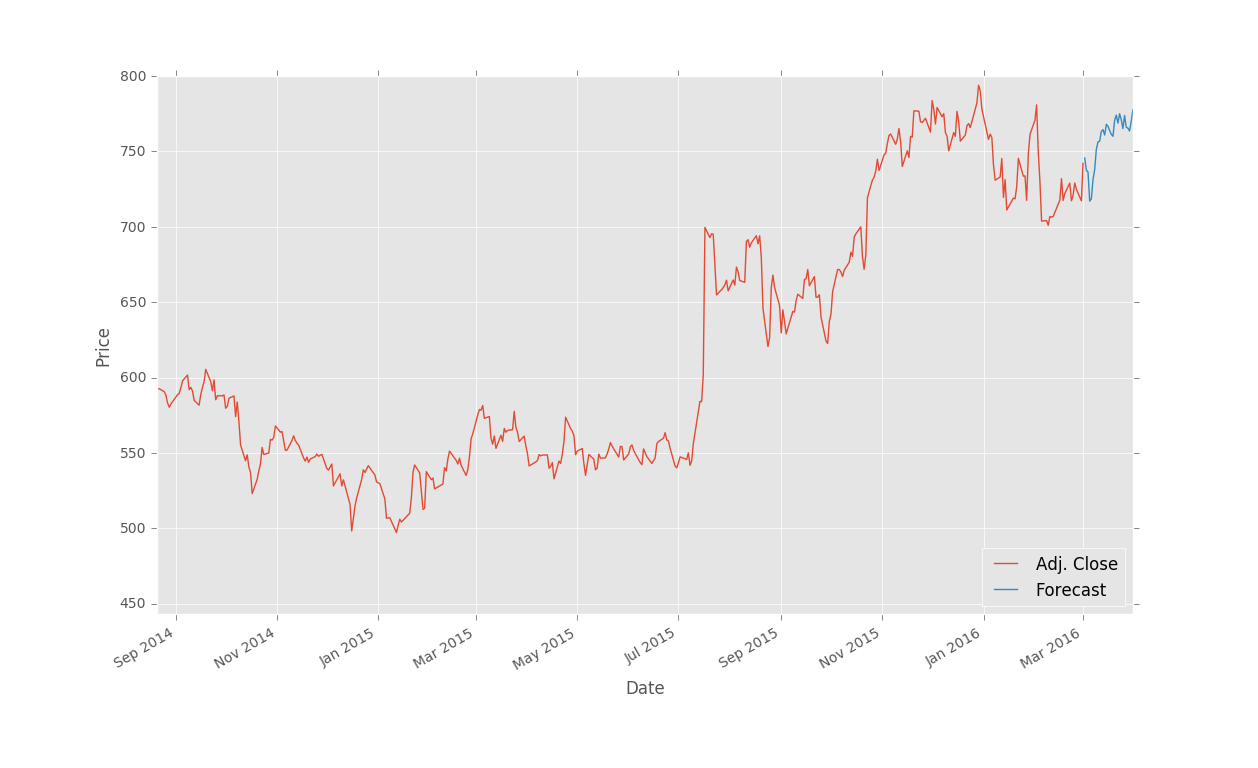import pickle

 with open('linearregression.pickle','wb') as f: pickle.dump(clf, f)

 pickle_in = open('linearregression.pickle','rb') clf = pickle.load(pickle_in)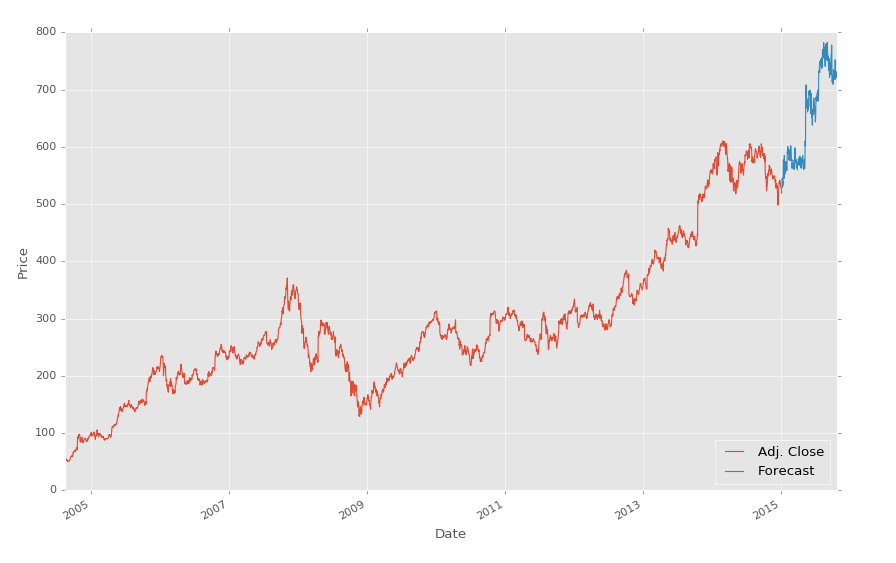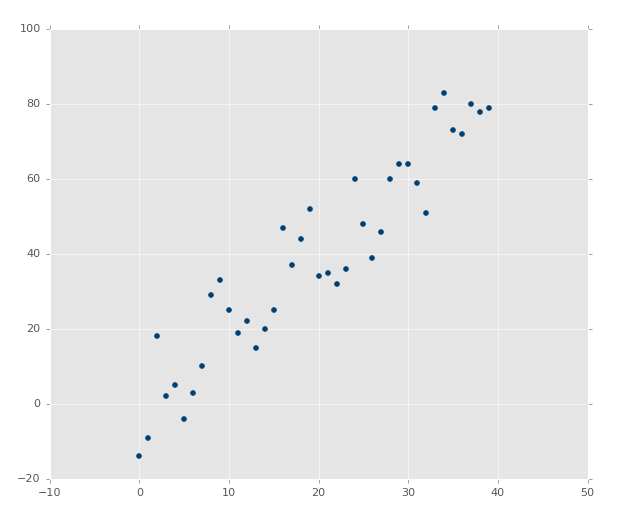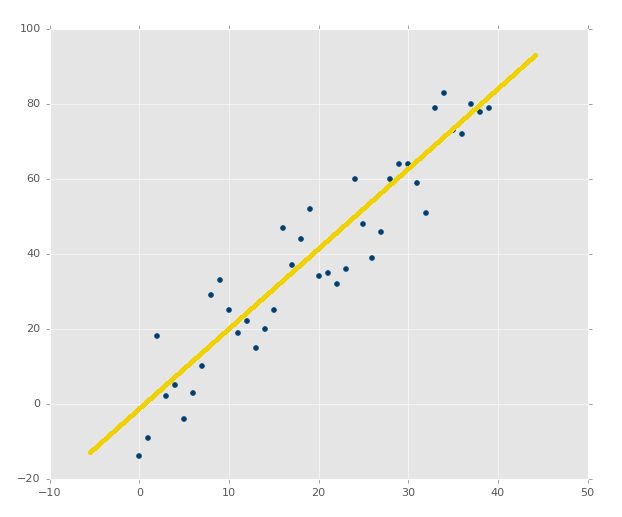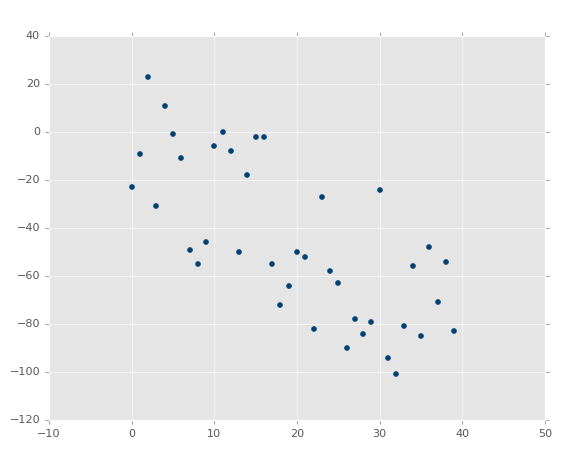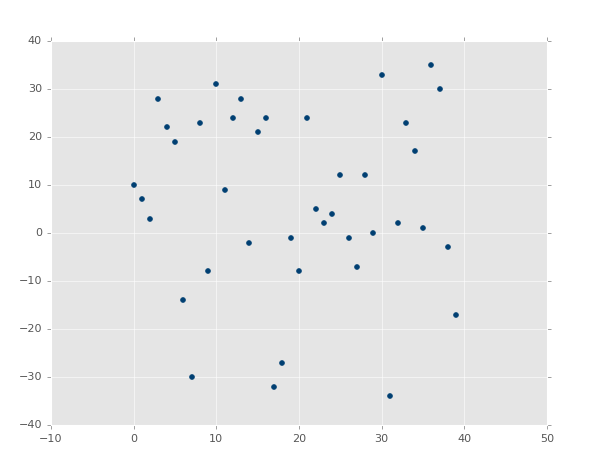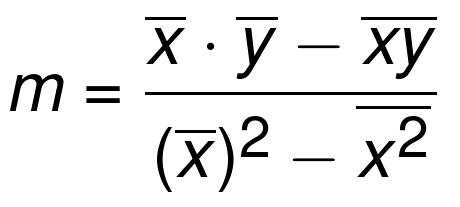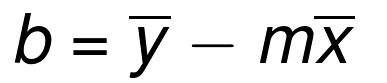from statistics import mean import numpy as np

 xs = [1,2,3,4,5] ys = [5,4,6,5,6]

 xs = np.array([1,2,3,4,5], dtype=np.float64) ys = np.array([5,4,6,5,6], dtype=np.float64)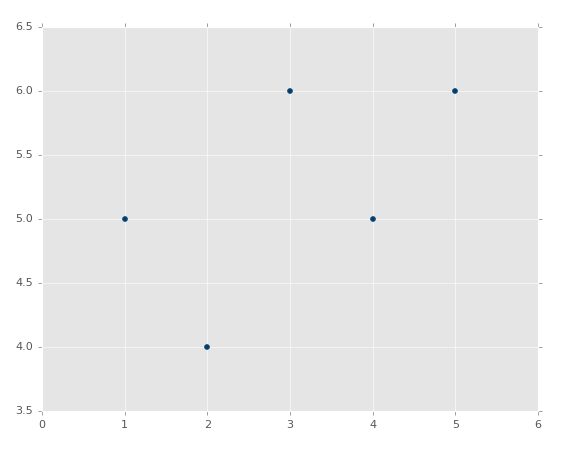def best_fit_slope(xs,ys): return m m = best_fit_slope(xs,ys)

 def best_fit_slope(xs,ys): m = (mean(xs) * mean(ys)) return m

 def best_fit_slope(xs,ys): m = ( (mean(xs)*mean(ys)) - mean(xs*ys) ) return m

 def best_fit_slope(xs,ys): m = ( ((mean(xs)*mean(ys)) - mean(xs*ys)) / (mean(xs)**2)) return m

 def best_fit_slope(xs,ys): m = (((mean(xs)*mean(ys)) - mean(xs*ys)) / ((mean(xs)**2) - mean(xs*xs))) return m

 from statistics import mean import numpy as np xs = np.array([1,2,3,4,5], dtype=np.float64) ys = np.array([5,4,6,5,6], dtype=np.float64) def best_fit_slope(xs,ys): m = (((mean(xs)*mean(ys)) - mean(xs*ys)) / ((mean(xs)**2) - mean(xs**2))) return m m = best_fit_slope(xs,ys) print(m) # 0.3

 from statistics import mean import numpy as np xs = np.array([1,2,3,4,5], dtype=np.float64) ys = np.array([5,4,6,5,6], dtype=np.float64) def best_fit_slope(xs,ys): m = (((mean(xs)*mean(ys)) - mean(xs*ys)) / ((mean(xs)*mean(xs)) - mean(xs*xs))) return m m = best_fit_slope(xs,ys) print(m)def best_fit_slope_and_intercept(xs,ys): m = (((mean(xs)*mean(ys)) - mean(xs*ys)) / ((mean(xs)*mean(xs)) - mean(xs*xs))) b = mean(ys) - m*mean(xs) return m, b

 best_fit_slope_and_intercept(xs,ys)

 from statistics import mean import numpy as np xs = np.array([1,2,3,4,5], dtype=np.float64) ys = np.array([5,4,6,5,6], dtype=np.float64) def best_fit_slope_and_intercept(xs,ys): m = (((mean(xs)*mean(ys)) - mean(xs*ys)) / ((mean(xs)*mean(xs)) - mean(xs*xs))) b = mean(ys) - m*mean(xs) return m, b m, b = best_fit_slope_and_intercept(xs,ys) print(m,b) # 0.3, 4.3regression_line = [(m*x)+b for x in xs]

 regression_line = [] for x in xs: regression_line.append((m*x)+b)

 import matplotlib.pyplot as plt from matplotlib import style style.use('ggplot')

 plt.scatter(xs,ys,color='#003F72') plt.plot(xs, regression_line) plt.show()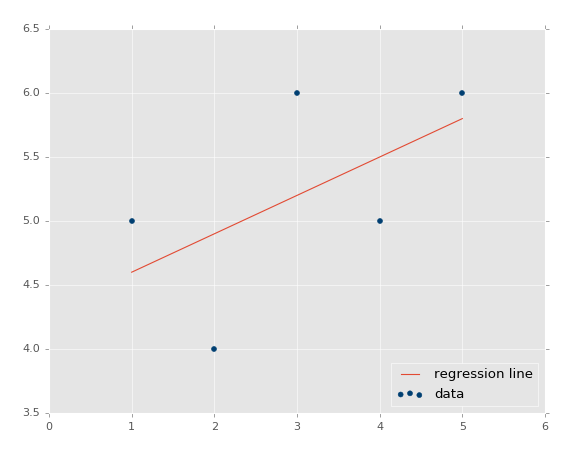predict_x = 7

 predict_y = (m*predict_x)+b print(predict_y) # 6.4

 predict_x = 7 predict_y = (m*predict_x)+b plt.scatter(xs,ys,color='#003F72',label='data') plt.plot(xs, regression_line, label='regression line') plt.legend(loc=4) plt.show()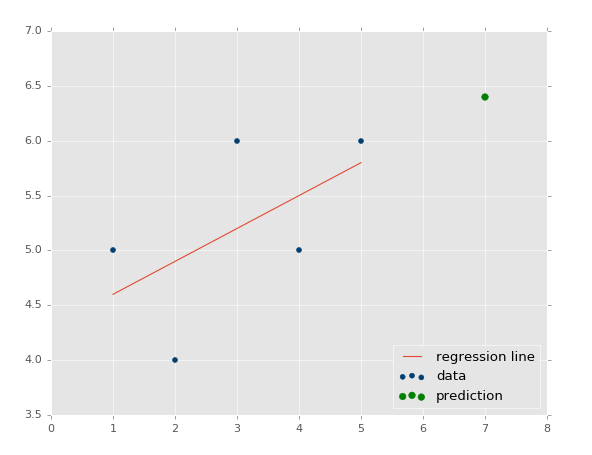R 平方和判定系数原理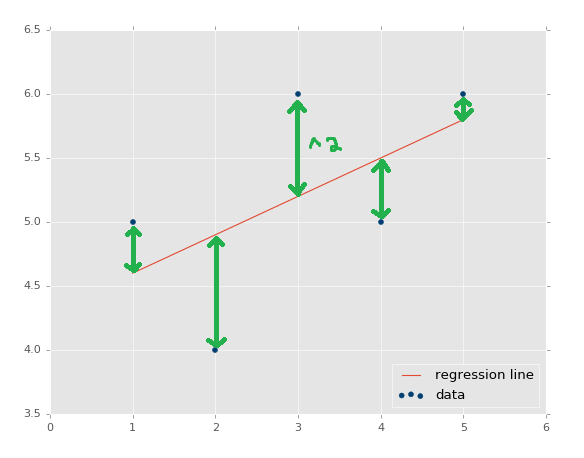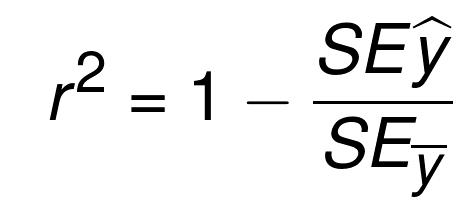y_hat = x * m + b r_sq = 1 - np.sum((y - y_hat) ** 2) / np.sum((y - y.mean()) ** 2)

 def squared_error(ys_orig,ys_line): return sum((ys_line - ys_orig) * (ys_line - ys_orig))

 def coefficient_of_determination(ys_orig,ys_line): y_mean_line = [mean(ys_orig) for y in ys_orig] squared_error_regr = squared_error(ys_orig, ys_line) squared_error_y_mean = squared_error(ys_orig, y_mean_line) return 1 - (squared_error_regr/squared_error_y_mean)

 from statistics import mean import numpy as np import matplotlib.pyplot as plt from matplotlib import style style.use('ggplot') xs = np.array([1,2,3,4,5], dtype=np.float64) ys = np.array([5,4,6,5,6], dtype=np.float64) def best_fit_slope_and_intercept(xs,ys): m = (((mean(xs)*mean(ys)) - mean(xs*ys)) / ((mean(xs)*mean(xs)) - mean(xs*xs))) b = mean(ys) - m*mean(xs) return m, b def squared_error(ys_orig,ys_line): return sum((ys_line - ys_orig) * (ys_line - ys_orig)) def coefficient_of_determination(ys_orig,ys_line): y_mean_line = [mean(ys_orig) for y in ys_orig] squared_error_regr = squared_error(ys_orig, ys_line) squared_error_y_mean = squared_error(ys_orig, y_mean_line) return 1 - (squared_error_regr/squared_error_y_mean) m, b = best_fit_slope_and_intercept(xs,ys) regression_line = [(m*x)+b for x in xs] r_squared = coefficient_of_determination(ys,regression_line) print(r_squared) # 0.321428571429 ##plt.scatter(xs,ys,color='#003F72',label='data') ##plt.plot(xs, regression_line, label='regression line') ##plt.legend(loc=4) ##plt.show()

 def create_dataset(hm,variance,step=2,correlation=False): return np.array(xs, dtype=np.float64),np.array(ys,dtype=np.float64)

1.hm（how much）：这是生成多少个数据点。例如我们可以选择 10，或者一千万。

2.variance：决定每个数据点和之前的数据点相比，有多大变化。变化越大，就越不紧密。

3.step：每个点距离均值有多远，默认为 2。

4.correlation：可以为False、pos或者neg，决定不相关、正相关和负相关。

 def create_dataset(hm,variance,step=2,correlation=False): val = 1 ys = [] for i in range(hm): y = val + random.randrange(-variance,variance) ys.append(y)

 def create_dataset(hm,variance,step=2,correlation=False): val = 1 ys = [] for i in range(hm): y = val + random.randrange(-variance,variance) ys.append(y) if correlation and correlation == 'pos': val+=step elif correlation and correlation == 'neg': val-=step

 def create_dataset(hm,variance,step=2,correlation=False): val = 1 ys = [] for i in range(hm): y = val + random.randrange(-variance,variance) ys.append(y) if correlation and correlation == 'pos': val+=step elif correlation and correlation == 'neg': val-=step xs = [i for i in range(len(ys))] return np.array(xs, dtype=np.float64),np.array(ys,dtype=np.float64)

 xs, ys = create_dataset(40,40,2,correlation='pos')

 from statistics import mean import numpy as np import random import matplotlib.pyplot as plt from matplotlib import style style.use('ggplot') def create_dataset(hm,variance,step=2,correlation=False): val = 1 ys = [] for i in range(hm): y = val + random.randrange(-variance,variance) ys.append(y) if correlation and correlation == 'pos': val+=step elif correlation and correlation == 'neg': val-=step xs = [i for i in range(len(ys))] return np.array(xs, dtype=np.float64),np.array(ys,dtype=np.float64) def best_fit_slope_and_intercept(xs,ys): m = (((mean(xs)*mean(ys)) - mean(xs*ys)) / ((mean(xs)*mean(xs)) - mean(xs*xs))) b = mean(ys) - m*mean(xs) return m, b def coefficient_of_determination(ys_orig,ys_line): y_mean_line = [mean(ys_orig) for y in ys_orig] squared_error_regr = sum((ys_line - ys_orig) * (ys_line - ys_orig)) squared_error_y_mean = sum((y_mean_line - ys_orig) * (y_mean_line - ys_orig)) print(squared_error_regr) print(squared_error_y_mean) r_squared = 1 - (squared_error_regr/squared_error_y_mean) return r_squared xs, ys = create_dataset(40,40,2,correlation='pos') m, b = best_fit_slope_and_intercept(xs,ys) regression_line = [(m*x)+b for x in xs] r_squared = coefficient_of_determination(ys,regression_line) print(r_squared) plt.scatter(xs,ys,color='#003F72', label = 'data') plt.plot(xs, regression_line, label = 'regression line') plt.legend(loc=4) plt.show()xs, ys = create_dataset(40,10,2,correlation='pos')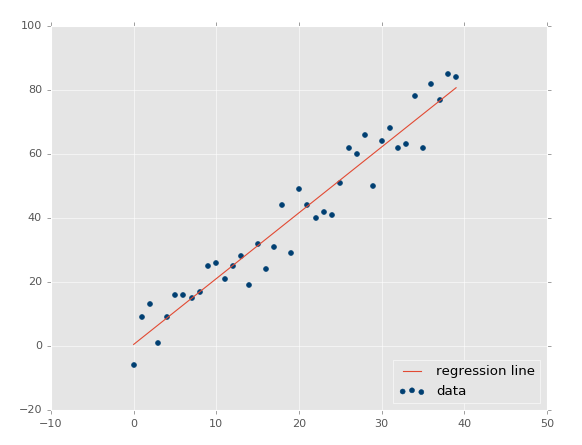xs, ys = create_dataset(40,10,2,correlation='neg')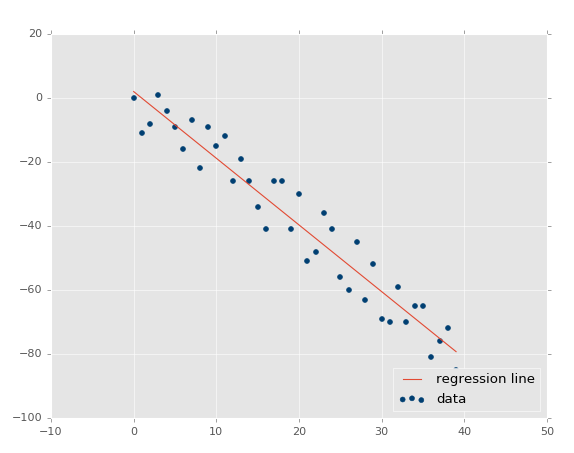R 平方值是 0.930242442156，跟之前一样好，由于它们参数相同，只是方向不同。

 xs, ys = create_dataset(40,10,2,correlation=False)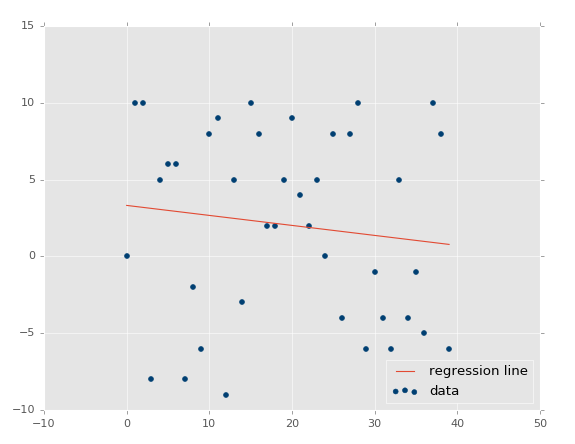2728 次浏览  评价: 好 中 差订阅捐助
 相关文章 手机软件测试用例设计实践 手机客户端UI测试分析 iPhone消息推送机制实现与探讨 Android手机开发（一）
 相关文档 Android_UI官方设计教程 手机开发平台介绍 android拍照及上传功能 Android讲义智能手机开发
 相关课程 Android高级移动应用程序 Android系统开发 Android应用开发 手机软件测试